Home » A Gentle Introduction to Handling a Non-Stationary Time Series in Python

# A Gentle Introduction to Handling a Non-Stationary Time Series in Python

## Introduction

What do these applications have in common: predicting the electricity consumption of a household for the next three months, estimating traffic on roads at certain periods, and predicting the price at which a stock will trade on the New York Stock Exchange?

They all fall under the concept of time series data! You cannot accurately predict any of these results without the ‘time’ component. And as more and more data is generated in the world around us, time series forecasting keeps becoming an ever more critical technique for a data scientist to master.

But time series is a complex topic with multiple facets at play simultaneously.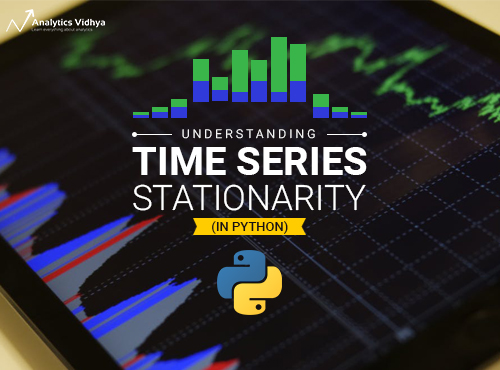For starters, making the time series stationary is critical if we want the forecasting model to work well. Why? Because most of the data you collect will have non-stationary trends. And if the spikes are erratic how can you be sure the model will work properly?

1. A Short Introduction to Stationarity
3. Methods to Check Stationarity
2. KPSS Test
4. Types of Stationarity
1. Strict Stationary
2. Trend Stationary
3. Difference Stationary
5. Making a Time Series Stationary
1. Differencing
2. Seasonal Differencing
3. Log transform

## 1. Introduction to Stationarity

‘Stationarity’ is one of the most important concepts you will come across when working with time series data. A stationary series is one in which the properties – mean, variance and covariance, do not vary with time.

Let us understand this using an intuitive example. Consider the three plots shown below: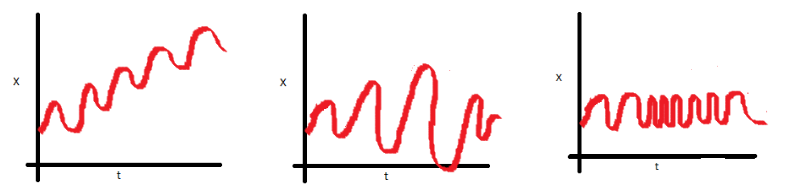• In the first plot, we can clearly see that the mean varies (increases) with time which results in an upward trend. Thus, this is a non-stationary series. For a series to be classified as stationary, it should not exhibit a trend.
• Moving on to the second plot, we certainly do not see a trend in the series, but the variance of the series is a function of time. As mentioned previously, a stationary series must have a constant variance.
• If you look at the third plot, the spread becomes closer as the time increases, which implies that the covariance is a function of time.

The three examples shown above represent non-stationary time series. Now look at a fourth plot: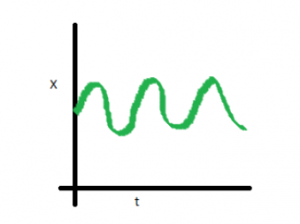In this case, the mean, variance and covariance are constant with time. This is what a stationary time series looks like.

Think about this for a second – predicting future values using which of the above plots would be easier? The fourth plot, right? Most statistical models require the series to be stationary to make effective and precise predictions.

So to summarize, a stationary time series is the one for which the properties (namely mean, variance and covariance) do not depend on time. In the next section we will cover various methods to check if the given series is stationary or not.

In this and the next few sections, I will be introducing methods to check the stationarity of time series data and the techniques required to deal with any non-stationary series. I have also provided the python code for applying each technique. You can download the dataset we’ll be using from this link: AirPassengers.

Before we go ahead and analyze our dataset, let’s load and preprocess the data first.

```#loading important libraries
import pandas as pd
import matplotlib.pyplot as plt
%matplotlib inline

#preprocessing
train.timestamp = pd.to_datetime(train.Month , format = '%Y-%m')
train.index = train.timestamp
train.drop('Month',axis = 1, inplace = True)

#looking at the first few rows

#Passengers
Month
1949-01-01 112
1949-02-01 118
1949-03-01 132
1949-04-01 129
1949-05-01 121

Looks like we are good to go!

## 3. Methods to Check Stationarity

The next step is to determine whether a given series is stationary or not and deal with it accordingly. This section looks at some common methods which we can use to perform this check.

### Visual test

Consider the plots we used in the previous section. We were able to identify the series in which mean and variance were changing with time, simply by looking at each plot. Similarly, we can plot the data and determine if the properties of the series are changing with time or not.

`train['#Passengers'].plot()`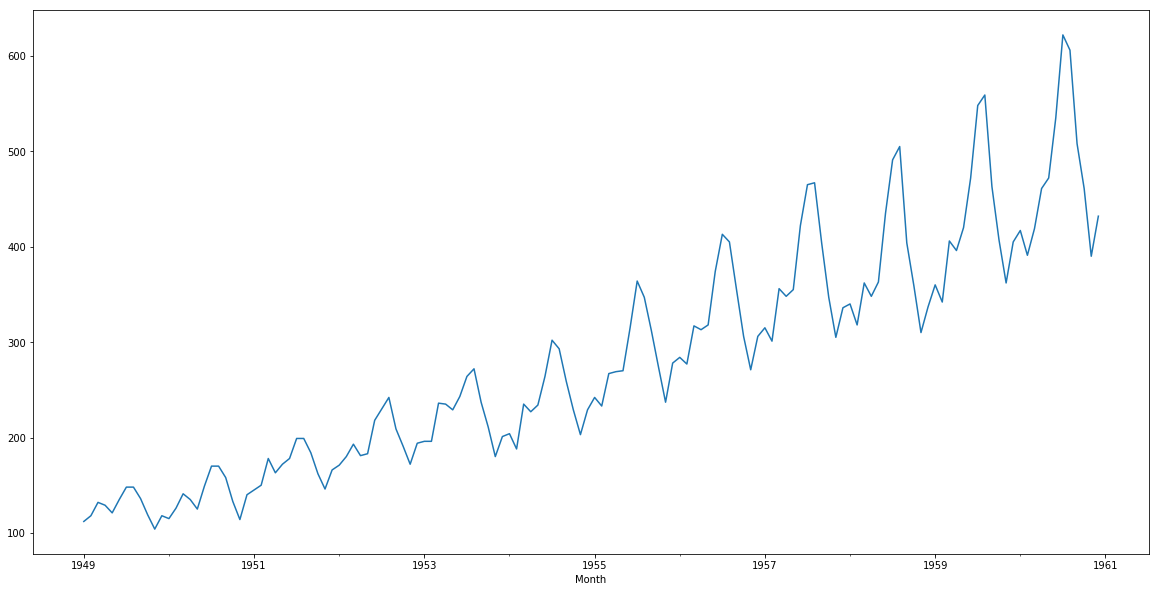Although its very clear that we have a trend (varying mean) in the above series, this visual approach might not always give accurate results. It is better to confirm the observations using some statistical tests.

### Statistical test

Instead of going for the visual test, we can use statistical tests like the unit root stationary tests. Unit root indicates that the statistical properties of a given series are not constant with time, which is the condition for stationary time series. Here is the mathematics explanation of the same :

Suppose we have a time series :

yt = a*yt-1 + ε t

where yt is the value at the time instant t and ε t is the error term. In order to calculate yt we need the value of yt-1, which is :

yt-1 = a*yt-2 + ε t-1

If we do that for all observations, the value of yt will come out to be:

yt = an*yt-n + Σεt-i*ai

If the value of a is 1 (unit) in the above equation, then the predictions will be equal to the yt-n and sum of all errors from t-n to t, which means that the variance will increase with time. This is knows as unit root in a time series. We know that for a stationary time series, the variance must not be a function of time. The unit root tests check the presence of unit root in the series by checking if value of a=1. Below are the two of the most commonly used unit root stationary tests:

#### ADF (Augmented Dickey Fuller) Test

The Dickey Fuller test is one of the most popular statistical tests. It can be used to determine the presence of unit root in the series, and hence help us understand if the series is stationary or not. The null and alternate hypothesis of this test are:

Null Hypothesis: The series has a unit root (value of a =1)

Alternate Hypothesis: The series has no unit root.

If we fail to reject the null hypothesis, we can say that the series is non-stationary. This means that the series can be linear or difference stationary (we will understand more about difference stationary in the next section).

Python code:

```#define function for ADF test
#Perform Dickey-Fuller test:
print ('Results of Dickey-Fuller Test:')
dfoutput = pd.Series(dftest[0:4], index=['Test Statistic','p-value','#Lags Used','Number of Observations Used'])
for key,value in dftest.items():
dfoutput['Critical Value (%s)'%key] = value
print (dfoutput)

#apply adf test on the series

Results of ADF test: The ADF tests gives the following results – test statistic, p value and the critical value at 1%, 5% , and 10% confidence intervals. The results of our test for this particular series are:

```Results of Dickey-Fuller Test:
Test Statistic                   0.815369
p-value                          0.991880
#Lags Used                      13.000000
Number of Observations Used    130.000000
Critical Value (1%)             -3.481682
Critical Value (5%)             -2.884042
Critical Value (10%)            -2.578770
dtype: float64```

Test for stationarity: If the test statistic is less than the critical value, we can reject the null hypothesis (aka the series is stationary). When the test statistic is greater than the critical value, we fail to reject the null hypothesis (which means the series is not stationary).

In our above example, the test statistic > critical value, which implies that the series is not stationary. This confirms our original observation which we initially saw in the visual test.

#### 2 . KPSS (Kwiatkowski-Phillips-Schmidt-Shin) Test

KPSS is another test for checking the stationarity of a time series (slightly less popular than the Dickey Fuller test). The null and alternate hypothesis for the KPSS test are opposite that of the ADF test, which often creates confusion.

The authors of the KPSS test have defined the null hypothesis as the process is trend stationary, to an alternate hypothesis of a unit root series. We will understand the trend stationarity in detail in the next section. For now, let’s focus on the implementation and see the results of the KPSS test.

Null Hypothesis: The process is trend stationary.

Alternate Hypothesis: The series has a unit root (series is not stationary).

Python code:

```#define function for kpss test
from statsmodels.tsa.stattools import kpss
#define KPSS
def kpss_test(timeseries):
print ('Results of KPSS Test:')
kpsstest = kpss(timeseries, regression='c')
kpss_output = pd.Series(kpsstest[0:3], index=['Test Statistic','p-value','Lags Used'])
for key,value in kpsstest.items():
kpss_output['Critical Value (%s)'%key] = value
print (kpss_output)```

Results of KPSS test: Following are the results of the KPSS test – Test statistic, p-value, and the critical value at 1%, 2.5%,  5%, and 10% confidence intervals. For the air passengers dataset, here are the results:

```Results of KPSS Test:
Test Statistic            1.052175
p-value                   0.010000
Lags Used                14.000000
Critical Value (10%)      0.347000
Critical Value (5%)       0.463000
Critical Value (2.5%)     0.574000
Critical Value (1%)       0.739000
dtype: float64
```

Test for stationarity: If the test statistic is greater than the critical value, we reject the null hypothesis (series is not stationary). If the test statistic is less than the critical value, if fail to reject the null hypothesis (series is stationary). For the air passenger data, the value of the test statistic is greater than the critical value at all confidence intervals, and hence we can say that the series is not stationary.

I usually perform both the statistical tests before I prepare a model for my time series data. It once happened that both the tests showed contradictory results. One of the tests showed that the series is stationary while the other showed that the series is not! I got stuck at this part for hours, trying to figure out how is this possible. As it turns out, there are more than one type of stationarity.

So in summary, the ADF test has an alternate hypothesis of linear or difference stationary, while the KPSS test identifies trend-stationarity in a series.

## 3. Types of Stationarity

Let us understand the different types of stationarities and how to interpret the results of the above tests.

• Strict Stationary: A strict stationary series satisfies the mathematical definition of a stationary process. For a strict stationary series, the mean, variance and covariance are not the function of time. The aim is to convert a non-stationary series into a strict stationary series for making predictions.
• Trend Stationary: A series that has no unit root but exhibits a trend is referred to as a trend stationary series. Once the trend is removed, the resulting series will be strict stationary. The KPSS test classifies a series as stationary on the absence of unit root. This means that the series can be strict stationary or trend stationary.
• Difference Stationary: A time series that can be made strict stationary by differencing falls under difference stationary. ADF test is also known as a difference stationarity test.

It’s always better to apply both the tests, so that we are sure that the series is truly stationary. Let us look at the possible outcomes of applying these stationary tests.

• Case 1: Both tests conclude that the series is not stationary -> series is not stationary
• Case 2: Both tests conclude that the series is stationary -> series is stationary
• Case 3: KPSS = stationary and ADF = not stationary  -> trend stationary, remove the trend to make series strict stationary
• Case 4: KPSS = not stationary and ADF = stationary -> difference stationary, use differencing to make series stationary

## 4. Making a Time Series Stationary

Now that we are familiar with the concept of stationarity and its different types, we can finally move on to actually making our series stationary. Always keep in mind that in order to use time series forecasting models, it is necessary to convert any non-stationary series to a stationary series first.

### Differencing

In this method, we compute the difference of consecutive terms in the series. Differencing is typically performed to get rid of the varying mean. Mathematically, differencing can be written as:

yt = yt – y(t-1)

where yt is the value at a time t

Applying differencing on our series and plotting the results:

```train['#Passengers_diff'] = train['#Passengers'] - train['#Passengers'].shift(1)
train['#Passengers_diff'].dropna().plot()```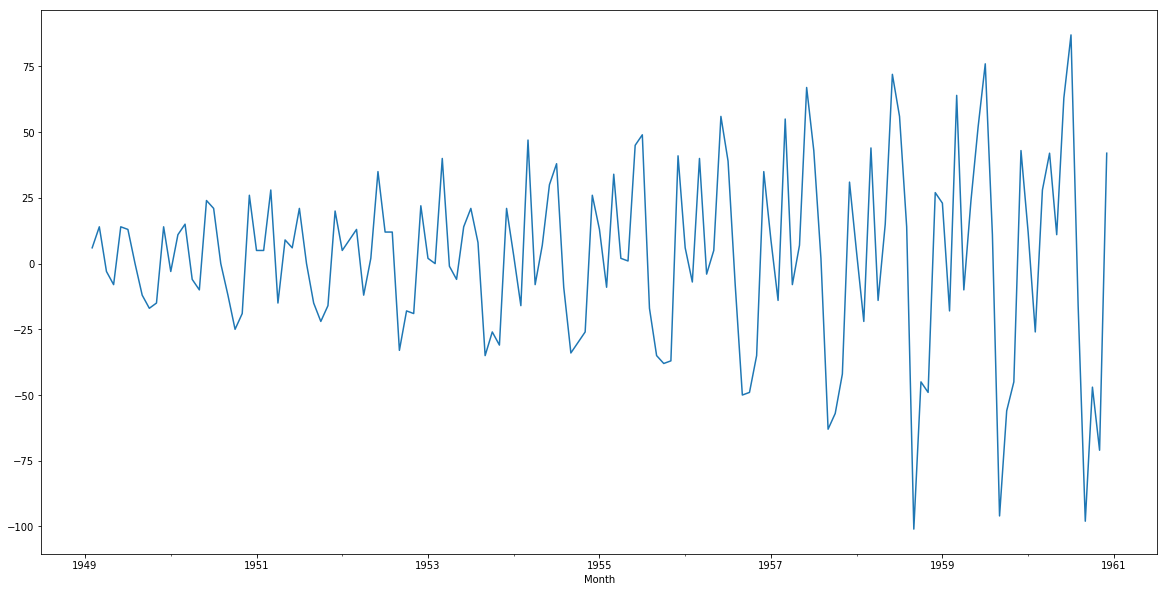### Seasonal Differencing

In seasonal differencing, instead of calculating the difference between consecutive values, we calculate the difference between an observation and a previous observation from the same season. For example, an observation taken on a Monday will be subtracted from an observation taken on the previous Monday. Mathematically it can be written as:

yt = yt – y(t-n)

```n=7
train['#Passengers_diff'] = train['#Passengers'] - train['#Passengers'].shift(n)```

### Transformation

Transformations are used to stabilize the non-constant variance of a series. Common transformation methods include power transform, square root, and log transform. Let’s do a quick log transform and differencing on our air passenger dataset:

```train['#Passengers_log'] = np.log(train['#Passengers'])
train['#Passengers_log_diff'] = train['#Passengers_log'] - train['#Passengers_log'].shift(1)
train['#Passengers_log_diff'].dropna().plot()```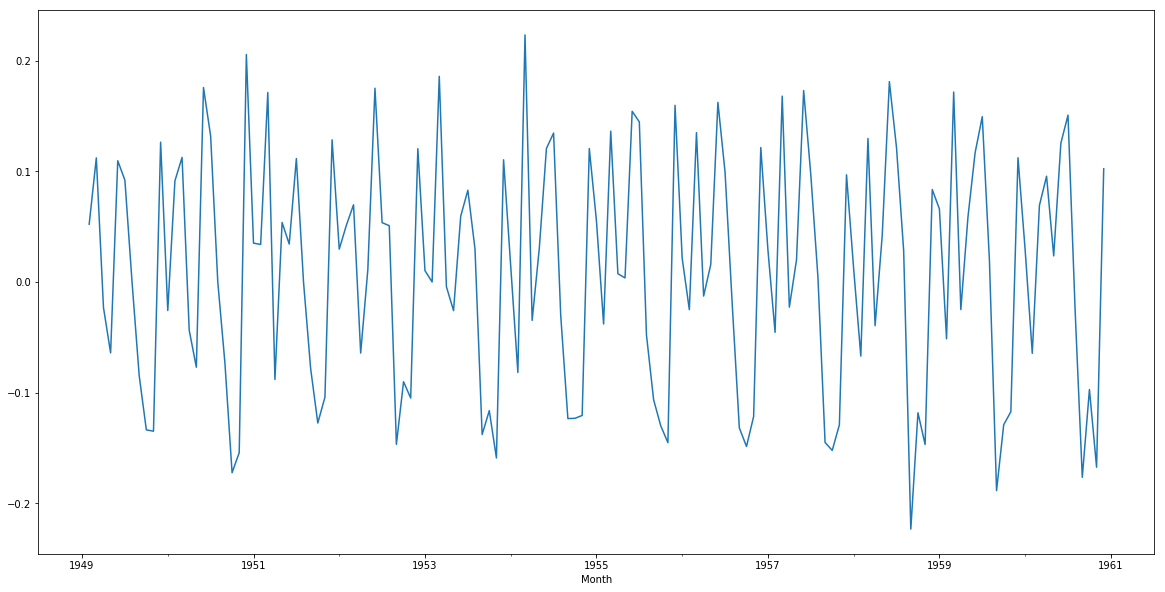As you can see, this plot is a significant improvement over the previous plots. You can use square root or power transformation on the series and see if they come up with better results. Feel free to share your findings in the comments section below!

## End Notes

In this article we covered different methods that can be used to check the stationarity of a time series. But the buck doesn’t stop here. The next step is to apply a forecasting model on the series we obtained. You can refer to the following article to build such a model: Beginner’s Guide to Time Series Forecast.

You can connect with me in the comments section below if you have any questions or feedback on this article.

You can also read this article on our Mobile APP•Carl says:

Great post. For differencing, you can also use the diff method on pandas Series and DataFrame objects.

•Aishwarya Singh says:

Thanks Carl!

•Miguel says:

I will call it “A Great Introduction to Handling a Non-Stationary Time Series in Python”. Thanks Aishwarya

•Aishwarya Singh says:

Thank you Miguel!

•Shreyansh says:

Hi Aishwarya, which test would be better to check the stationarity, ADF Test or the KPSS test?

•Aishwarya Singh says:

Hi Shreyansh,

It’s preferred to apply both the tests to check if the series is stationary. In case the tests give contradictory results, you would have to deal with the dataset accordingly (remove trend or perform differencing operation based on the results)

•Alex says:

Hi there. I recently come across few articles on transformation of non stationary data and mentioned quite extensive use of the Box Cox transformation technique as an alternative to the log transformation. What is you oppinion on such the approach and what the major use case differences between the Box Cox and log.
Thanks
Alex

•Aishwarya Singh says:

Hi Alex,

Log transform is a derivative of box cox transform. If you give the lambda value as 0, it will perform a log transform. The main difference is that unlike log transform, box cox is not restricted to one value. This link shows a table for the transformations performed for different lambda values in box cox.

•John says:

Thank you for the great article. Between Differencing / Seasonal Differencing & Log transformation, looks like log transformation provides the best result. Could that be the default one to do for most time series data.
Thank you

•Aishwarya Singh says:

Hi John,

Both the transformations are for different purposes. Differencing removes the trend present in the series while log transformation is for handling high variance in the series. Based on the dataset, you can use any one or both of the methods to make the series stationary.

•vignesh says:

Case 3: KPSS = stationary and ADF = not stationary -> trend stationary, remove the trend to make series strict stationary
Case 4: KPSS = not stationary and ADF = stationary -> difference stationary, use differencing to make series stationary

In the above two points, you have stated that in case 3, we should remove trend and in case 4, we should do differencing, and now in the reply to JOHN, you are telling that differencing removes trend in the series.

so whether, it is case 3 or case 4, we have to apply differencing?

Thanks

•Aishwarya Singh says:

Differencing removes the linear trend in the series. If you have an exponential trend or some other pattern, then differencing would not be the best approach. That’s the major difference between case 3 and case 4.

•Henry says:

Good article. Quick question.
Once we make it stationary, can we model this as a regression problem using month / Yr and the transformed feature (difference or difference) as the target variable?
Best,

•Aishwarya Singh says:

Hi Henry,

I haven’t tried it before but I see no reason why this cannot be treated as a regression problem. You’ll just have to perform reverse of the transformations after you get the results.

•Robert says:

Hi Aishwarya,

Im afraid that I don’t understand. What did you graph after you changes in the transform? Third line seems like it should be:

train[‘#Passengers_log’] = np.sqrt(train[‘#Passengers_log_diff’].dropna()).plot()

How can I invert this so I am graphing the original data

•Aishwarya Singh says:

Hey Robert,

Thanks for pointing it out, I have updated the code now. Please check.

•Robert says:

Ahh that is clearer, thank you Aishwarya.

I have read that the typical way to do this with np is something like this:

for column in endog:
min_nonzero = series[series

> 0].min()
series.loc[series

== 0, column] = min_nonzero - 0.00001
series

= np.log(series

).diff()

then, to invert, you should np.exp(train.append(y_pred).cumsum())

I'm having trouble with the inversion process though (see https://stackoverflow.com/questions/52951297/invert-a-log-transpose-for-plotting ) -- how do you invert your data to get the original curve?

•Aishwarya Singh says:

Hi Robert,

While inverting, make sure you follow the correct order of transform and differencing. Initially if you performed log and then differencing, use cumsum then exp. Secondly, if you could show the plot of the predictions without exp and differecning, it would help me understand the problem better.

•Davis David says:

Nice Article ever!!

•Aishwarya Singh says:

Thank you Davis!

•KRISHNAKUMAR MISHRA says:

Beautifully explained the different aspects involved.